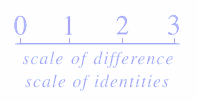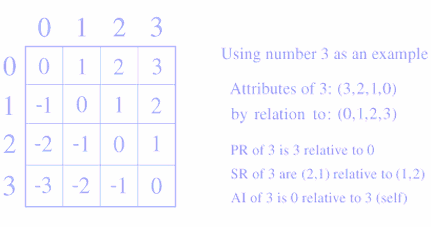# - Identity Defined -

```
```

Identity defines the fundamental nature of every thing, whether that thing is an objective physical entity or a subjective mental construct. To understand the nature of things we must first understand the nature of identity.

Exceeding the standard definition of identity as just self- similarity, the structure of identity expresses a duality of (1) self-similarity and (2) other-difference, for we can say that a thing (a part of all) is the same as itself if, and only if, we can say it is different than something else. As we shall see, these two aspects of identity create Absolute and Relative identity attributes. This then is the dual definition of identity:

DUAL DEFINITION OF IDENTITY

(1) ABSOLUTE: zero difference; the unconditional nature of a thing that is not derived from external relation; the product of internal self-similarity. Example: She must be Mary since she is the same as Mary.

(2) RELATIVE: nonzero difference; the conditional nature of a thing, n, derived from the difference between n and not(n); the product of external other-difference. Example: Smith is best since he scored more than the others.

The identity of a thing is simultaneously (1) and (2). Example: the difference between 4 and 4 is 0 (4 is the same as 4) because the difference between 4 and 0 is 4. So a thing, n, is defined at the same time by both its similarity to itself and by its difference from not(n); and thus difference (from zero difference to nonzero differences) defines the whole structure of identity.

SYNTHETIC IDENTITY ANALYSIS

Numbers are synthetic identities that serve as ideal subjects for the study of identity. It is because they are the ideal model of identity that numbers and their relations, while synthetic, are unparalleled in their ability to model the natural world.

"Treat the laws and relationships of integers like
those of the celestial bodies."
George Cantor

Identity structure is ideally expressed as a numeric scale of difference from zero to infinity. Zero is no difference, or similarity, and all other numbers to infinity are degrees of difference defined by their difference, or displacement, from zero.Each number-identity along the continuum is defined by its difference from zero (n - 0 = n), which defines the Primary Relative (PR) identity attribute of an identity. For example, 3 is 3 because 3 is 3 more then 0. A number n also has Secondary Relative (SR) identity attributes, which express the difference between n and other numbers that are nonzero. We can see this in the differentiation matrix below.

PR = Primary Relative identity attribute
SR = Secondary Relative identity attributes
AI = Absolute Identity attribute

Listed in the vertical column below each number on the top of the matrix below are its identity attributes as derived from relations to the numbers listed along the left side. The identity attributes derived from the relation of two numbers are found at their intersections:Example of SR attribute: 3 is 2 more than 1. "3 is 2 more" is a statement about the identity of 3 relative to 1, thus "2 more" is a SR identity attribute of 3. In the same way, "Smith is 2 feet taller" defines a SR identity attribute (+2ft) that belongs to Mr Smith relative to Mr Jones. Smith is also 4 feet shorter (-4ft) than an elephant just as 3 is also 6 less than 9. Therefore, a thing, n, has as many SR attributes as there are other things n can be related to, yet n only has one PR attribute, n.

PR = Primary Relative identity attribute
SR = Secondary Relative identity attributes
AI = Absolute Identity attribute

The AI attribute of each number (found at its intersection with itself in the table) and the AI of all numbers is zero. Therefore, 0 = (AI of 1) = (AI of 2) = (AI of 3) = 0. It is the relative identity attributes derived from holistic relations that define a thing as a unique subset of the whole. Self-similarity is Absolute identity, it is the "home base" of that which is "self," and, being a null relation, is equal to zero.

The zero of Absolute identity defines the Buddhist claim about identity, that the inherent (i.e., the absolute) self-nature of all things is void (i.e., is zero), and that what we think of as the thing is merely a product of conditional relations.

CONCLUSION

The analysis of synthetic numeric identities herein allows us to see and thereby prove: (a) that identity is conserved, expressed via the real numbers as a constant zero-sum, since, for example, n is always as much more than x as x is less than n; (b) that identity is therefore symmetrical; (c) that Relative identity is holistic, since all nonzero identity attributes are derived from other-difference; and (d) that the Absolute (nonrelative) identity of each and every thing equals zero.

WHY DIFFERENCE DEFINES IDENTITY

Impressed by the complex we overlook the simple, we associate simple with that which we must pass beyond in our quest for the ultimate understanding and truth. Yet, just as the complex structures of a fractal design come from an infinite repetition of a relatively simple primary form, infinite complexity comes from the primary simplicity of difference, from zero difference (similarity) to infinity...

The numbers along the real-number line express progressive displacement, or additional difference, relative to zero, the origin. Each number is its separation, displacement, or difference, from zero, thus n is exactly n more than 0. So the essential nature of an identity is a state of difference.

If there was no difference, there would be no numbers, and if there were no numbers, there would be no mathematics. Before you can build a mathematical statement, first you need numbers, which are states of difference. The same principle holds for physical things, which are also states of difference, such that no difference implies no things. So the fundamental nature of identity and thus of all things is that of difference, from zero difference to infinity...

Therefore, difference, expressed as subtraction, is unique among all mathematical operations in that only difference defines the primary causal structure of identity. The scale of difference, from zero to infinity defines the causal structure of identity, of what it is that makes a thing what it is, and thus the differentiation table is the template that defines the foundation of identity and existence.

```
```

(c) 1998 Ian Williams Goddard